Courses

# Short Answers Type Questions - Probability Class 9 Notes | EduRev

## Mathematics (Maths) Class 9

Created by: Full Circle

## Class 9 : Short Answers Type Questions - Probability Class 9 Notes | EduRev

The document Short Answers Type Questions - Probability Class 9 Notes | EduRev is a part of the Class 9 Course Mathematics (Maths) Class 9.
All you need of Class 9 at this link: Class 9

Question 1. If we throw a die, then the upper face shows 1 or 2; or 3 or 4; or 5 or 6. Suppose we throw a die 150 times and get 2 for 75 times. What is the probability of getting a ‘2’?
Solution:
Let E be the event of getting 2.

∴      P(E) = Number of favourable outcomes/ total number of trails

= (75/150) = (1/2) = 0.5

Question 2. A coin is tossed 200 times and is found that a tail comes up for 120 times. Find the probability of getting a tail.
Solution:
Number of trials = 200
Number of favourable outcomes = Number of getting a tail
= 120
Let the probability of getting a tail is P(E).

∴    P(E) = Number of favourable outcomes/ total number of trails = (120/200) = (6/10) = 0.6

Question 3. If a coin is tossed for a certain number of times. How many times the coin was tossed, if the probability of getting a head is 0.4 and it appeared up for 24 times?
Solution:
Number of favourable outcomes = 24
Let the total number of trials is n.

Here,               P(E) = Number of favourable outcomes/ total number of trails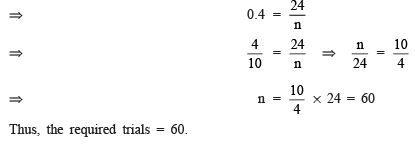.

Question 4. In a cricket match, if the probability P(E) of hitting the boundary is 0.3, then find the probability of not-hitting the boundary.
Solution:
Probability of hitting the boundary P(E) = 0.3
∴ Probability of not-hitting the boundary = 1 – P(E) = 1 – 0.3 = 0.7

Question 5. In a GK test a student was given 50 questions one by one. He gave the correct answer for 30 questions. Find the probability of giving correct answers.
Solution:
Total number of trials = 50
Number of favourable outcomes = Number of correct answers = 30
Let P(E) be the probability of giving correct answers.
∴           P(E) = Number of favourable outcomes/ total number of trails = (30/50) = (60/100) = 0.6

Question 1. Write ‘true’, ‘false’ or ‘ambiguous’ for each of the following statements: (i) All prime numbers are odd. (ii) Division by 0 is not possible. (iii) There are 8 days in a week. (iv) 2 + 3 = 5 (v) Raju is a poor boy. (vi) Cats can fly.
Solution:
(i) False (ii) True (iii) False (iv) True (v) Ambiguous (vi) False

Question 2. State whether the following statements are true or false: (i) The sum of the interior angles of a triangle is 360° (ii) The number 2 is the only even prime number. (iii) Every odd number is greater than 2. (iv) Every angle formed in semicircle is 90°. (v) When we divide a number by 0, we get 1. (vi) For every real number x, x2 = 2x.
Solution:
(i) False (ii) True (iii) False (iv ) True (v) False (vi ) False

Question 3. Define a (i) Theorem, (ii) Axiom and (iii) Conjecture.
Solution:
(i) Theorem: A mathematical statement which can be proved to be true.
(ii) Axiom: The statements which are assumed to be true and are taken to be true without proof.
(iii) Conjecture: A conjecture is a mathematical statement whose truth or falsity is yet to be established.

Question 4. Prove that the product of two even natural numbers is divisible by 16.
Solution:
Let the two even numbers be x and y.
Since, x is an even number, it is divisible by 2.
∴ x = 2m [where m is a natural number]
Also, y = 2n [where n is a natural number]
∴ xy = (2m) x (2n) = 4mn Since, 4mn is divisible by 2.
∴ xy is also divisible by 2.
Thus, xy is even.

Question 5. A coin is tossed 150 times and it is found that head comes 115 times and tail 35 times.
If a coin tossed at random, what is the probability of getting (i) a head (ii) a tail
Solution:
Here, total number of trials = 150

(i)  ∵ Number of heads as outcome = 115
∴ Probability of an event of getting a head = (115/150)= (23/30)

(ii) ∵ Number of tails as outcomes = 35
∴ Probability of an event of getting a tail = (35/150) = (7/30)

REMEMBER

(i) The probability of an event can be 0 to 1.
(ii) [Probability of the occurrence of an event] + [Probability of non-occurrence of that event] = 1
(iii) The sum of the probability of all the possible outcomes of a trial = 1

Question 6. A die is thrown 270 times and the outcomes are recorded as in the following table:

 Outcome 1 2 3 4 5 6 Frequency 36 45 33 18 75 63

If a die is thrown at random, find the probability of getting: (i) 1 (ii) 2 (iii) 3 (iv) 4 (v) 5 (vi) 6
Solution:
Here, total number of trials = 270
Let P(E1), P(E2), P(E3), P(E4), P(E5) and P(E6) be the probability of a throw and getting 1, 2, 3, 4, 5 and 6 respectively.
Now, (i) ∵ Number of events of getting 1 = 36
∴ Probability of a throw to get 1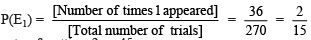(ii) ∵ Number of events of getting 2 = 45
∴ Probability of a throw to get 2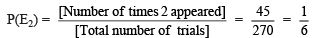(iii) ∵ Number of events of getting 3 = 33
∴ Probability of a throw to get 3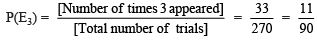(iv) ∵ Number of events of getting 4 = 18
∴ Probability of a throw to get 4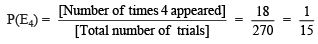(v) ∵ Number of events of getting 5 = 75
∴ Probability of a throw to get 5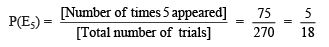(vi) ∵ Number of events of getting 6 = 63
∴ Probability of a throw to get 6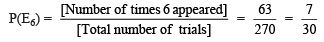Note: P(E1) + P(E2) + P(E3) + P(E4) + P(E5) + P(E6)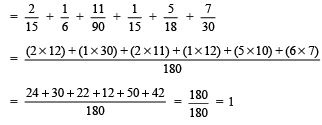,

,

,

,

,

,

,

,

,

,

,

,

,

,

,

,

,

,

,

,

,

;